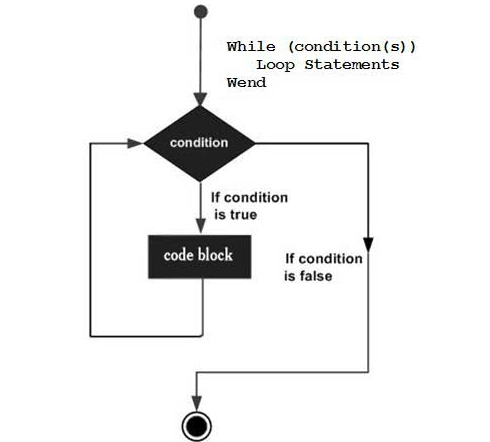#### While Wend Loop in Excel Vba

In this Excel Vba tutorial article, I will explain how to use the While Wend loop in Excel VBA Code.

## The loop

Loops are a powerful tool in Excel VBA that can be used to automate tasks and perform repetitive operations. One type of loop is the While Wend loop, which is used to execute a block of code as long as a certain condition is true. We use loops in Excel when we have to run several condition checks repeatedly in Excel VBA. Sometimes we use: if, end if loop which is a very simple and most used loop.

If loop:

If condition then

“Process if condition is true”

End if

Then we have a for loop if we know exactly how many times the loop must run:

For loop:

For I = 1 to N

Process.

Next i

In this loop, it will run the process for a N number of times.

Now sometimes we have a certain condition, so we must keep the loop running as many times as possible till the condition is not true. Here comes the While Wend loop:

Syntax:

While condition(s)

[statements 1]

[statements 2]

[statements n]

Wend

The condition is a Boolean expression that must evaluate to either True or False. If the condition evaluates to True, the block of code inside the loop will be executed. If the condition evaluates to False, the loop will end.

It will keep running till the condition is true:It’s important to make sure that the condition in the “While” loop will eventually become false, otherwise the loop will continue to execute forever, which is known as an infinite loop.

First, the While condition is tested.

• if the condition is FALSE, the program immediately jumps to the command immediately after the word Wend
• if the condition is TRUE, then all commands inside the loop (i.e., between the words Do While and Loop) are executed.

## Examples

### Basic While Wend Loop example

Sub While_wend_Example()

Dim countA: countA = 100

While countA < 110 ‘ Test value of Counter.

MsgBox “The Current Value of the Counter is : ” & countA

countA = countA + 1 ‘ Increment Counter.

Wend

End Sub

This loop will keep running till we have the condition true : countA value is less than 110.

So this loop will run 10 times:

Iteration1 : CountA = 100

Iteration2 : CountA = 101

Iteration3 : CountA = 102

Iteration4 : CountA = 103

Iteration5 : CountA = 104

Iteration6 : CountA = 105

Iteration7 : CountA = 106

Iteration8 : CountA = 107

Iteration9 : CountA = 108

Iteration10 : CountA = 109

For the next iteration, CountA = 110 so the condition will become false and the loop ends.

### Calculating the factorial of numbers in a While Wend Loop

Sub Factorial()

x = 0

y = 0

While x < 10

x = x+1

y = y*x

Wend

ActiveDocument.Content= “Factorial result of ” & x & ” = ” & y

End Sub

### While Wend Loop to calculate SUM in Excel.

Sub SumWhileWend()

n = InputBox(“Enter n value:”)

Dim j As Single

Dim i As Integer

i = 1

While (i <= n)

j = j + i

i = i + 1

Wend

MsgBox j

End Sub

You can also use the “Do While” loop in Excel VBA, which is similar to the “While” loop but with a slight difference in the way the condition is checked. The “Do While” loop checks the condition after the first iteration of the loop, while the “While” loop checks the condition before the first iteration of the loop.

It is important to make sure that the condition in the While Wend loop will eventually become False, otherwise the loop will continue to execute forever, which is known as an infinite loop.

Here are some additional tips for using While Wend loops:

• Use the `Do While` loop if you want the loop to execute at least once, even if the condition is initially False.
• Use the `Exit While` statement to exit the loop early if the condition is met.
• Use the `Continue` statement to skip the rest of the current iteration of the loop and go to the next iteration.

## Key Takeaways

• While Wend loops are used to execute a block of code as long as a certain condition is true.
• The syntax for a While Wend loop is `While condition [statements] Wend`.
• It is important to make sure that the condition in the While Wend loop will eventually become False, otherwise the loop will continue to execute forever.

## FAQ

• Q: What is the difference between a While Wend loop and a Do While loop?
• A: The main difference between a While Wend loop and a Do While loop is that the Do While loop checks the condition after the first iteration of the loop, while the While Wend loop checks the condition before the first iteration of the loop.
• Q: How can I avoid infinite loops?
• A: To avoid infinite loops, you need to make sure that the condition in the While Wend loop will eventually become False. You can do this by using a counter variable that is incremented or decremented each time the loop iterates.